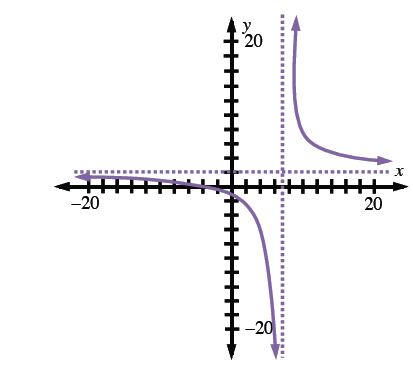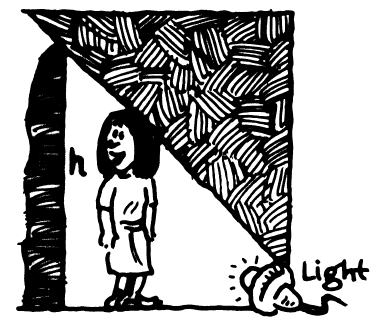### Home > PC > Chapter 6 > Lesson 6.3.2 > Problem6-127

6-127.

Graph $y=\frac { 2 x + 7 } { x - 7 }$.

Remember, fraction can’t have 0 in the denominator. Rewrite the function as a stretch and shift of $y=\frac{1}{x}$.

1. What is the domain?1. For each of the following questions, write the corresponding limit statement and evaluate it.

1. What happens to $y$ as $x$ gets close to $7$ and $x \gt 7$?

2. What happens to $y$ as $x$ gets close to $7$ and $x \lt 7$?

3. What happens to $y$ as $x$ approaches positive infinity?

4. What happens to $y$ as $x$ approaches negative infinity?